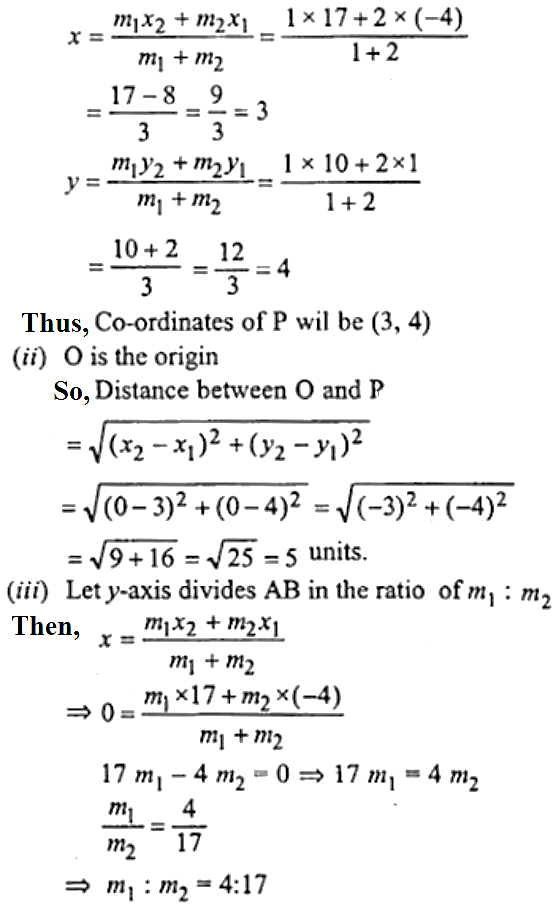Newbie

# (i) Write down the co-ordinates of the point P that divides the line joining A ( – 4, 1) and B (17, 10) in the ratio 1 : 2. (ii) Calculate the distance OP where 0 is the origin (iii) In what ratio does the y-axis divide the line AB?

• 0

This is the Important question of class 10 Based on Equation of a Straight Line Chapter of M.L Aggarwal book for ICSE BOARD.
Here you have to find the co-ordinates of a point that divides the line joining two coordinates in the given ratio. Solve this question. I have so many doubts.
This is the Question Number 39, Exercise 12.2 of M.L Aggarwal.

Share

1.